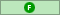Forumwarz is the first "Massively Single-Player" online RPG completely built around Internet culture.

You are currently looking at Flamebate, our community forums. Players can discuss the game here, strategize, and role play as their characters.

You need to be logged in to post and to see the uncensored versions of these forums.Help with math plz

# a dallop a d-aisy## Level 6 Camwhore

Plz hlp I hav a problem in math i dun understand plz hlp me here

let M(S) denote the monotone clbum generated by a set of sets S

i dun understand why ∀S S is a ring ∀A x∈M(S) ∃{A_n} (A_n⊂A_(n+1)∧S_n∈S∧(∪(S_n)=S)

plz explain this to me i dun understand why this is true, its a fact used for proving the uniqueness of extention of measure

# Zirolol?

# a dallop a d-aisy## Level 6 Camwhore

acutually i made a mistake plz forgiv me the actual statement i dun understand is (corections are bolded):

∀R R is a ring∀A A∈M(R )∃{A_n} (A_n⊂A_(n+1)∧A_n∈R∧(∪(A_n)=A)

thar i think i wroat it in proper formal language plz tell me why this is true

a dallop a daisy edited this message on 03/07/2008 8:40PM2

## Level 10 Troll

### “Pain in the ASCII”

[This message has randomly exploded, causing the “divide by 0” effect, and thus creating a pime taradox.]

Warpvader edited this message on 03/10/2008 9:52PM

# Opportunity8

Do a barrelroll.

# Harix2

## Level 10 Camwhore

### “Leave it to Cleavage”

The problem is that there are upside down “A”s in your statement…

# dreamserpent12

## Level 10 Camwhore

### “Leave it to Cleavage”

the upside down A just means “for all” LOLOL NOOB

# a dallop a d-aisy## Level 6 Camwhore

o its very simple

it means “for all rings R, for all A within M(R ), there exists a sequence of sets A_n which are elements of R such that their union is A.”

to see wat im talking about, here is an example in which it is implicitly stated:

http://www-maths.swan.ac.uk/staff/vl/c98.pdf go to page 18, theorem 4.2

Theorem 4.2 Let A be an algebra of sets, μ and ν two measures defined on the σ-algebra A_σ generated by A. Then μ ↾ A =  ν ↾ A implies μ = ν.

Proof. Choose S ∈ A_σ, then S=lim (n⇒∞) S_n, S_n ∈A, for A_σ=M(A ). Using continuity of measure, one has

μ(S) = lim (n⇒∞) μ(S_n) = lim (n⇒∞) ν(S_n) = ν(S).

a dallop a daisy edited this message on 03/07/2008 9:01PM

# Miggle2

# Skyreal8

## Level 24 Camwhore

### “The Lady is a Tramp”

a dallop a daisy Posted:

acutually i made a mistake plz forgiv me the actual statement i dun understand is (corections are bolded):

∀R R is a ring∀A A∈M(R )∃{A_n} (A_n⊂A_(n+1)∧A_n∈R∧(∪(A_n)=A)

thar i think i wroat it in proper formal language plz tell me why this is true

(A_n⊂A_(n+1)∧A_n∈R∧(∪(A_n)=A) has me pretty confused.

# a dallop a d-aisy## Level 6 Camwhore

Skyreal Posted:

(A_n⊂A_(n+1)∧A_n∈R∧(∪(A_n)=A) has me pretty confused.

it means the sequence of sets A_n is increasing and each A_n is in the ring R and the union of it is A.

# PhineasPoe8

## Level 35 Troll

### You got a smudge there Phin... oh wait thats Trouts feces

Drop the clbum before it does any more damage.

# VIVa-ChiCk1

The game

# Balloon28

## Level 35 Camwhore

### Inflate my ovaries until they pop out of me and float away

The only thing I remember from math was not to use my teeth.

# crackednut## Level 13 Troll

### “Pain in the ASCII”

T∀R R is a ring⇒∀A A∈M(R )⇒∃{A_n} (A_n⊂A_(n+1)∧A_n∈R∧(∪(A_n)=A)∀R R is a ring⇒∀A A∈M(R )⇒∃{A_n} (A_n⊂A_(n+1)∧A_n∈R∧(∪(A_n)=A)∀R R is a ring⇒∀A A∈M(R )⇒∃{A_n} (A_n⊂A_(n+1)∧A_n∈R∧(∪(A_n)=A)∀R R is a ring⇒∀A A∈M(R )⇒∃{A_n} (A_n⊂A_(n+1)∧A_n∈R∧(∪(A_n)=A)∀R R is a ring⇒∀A A∈M(R )⇒∃{A_n} (A_n⊂A_(n+1)∧A_n∈R∧(∪(A_n)=A)∀R R is a ring⇒∀A A∈M(R )⇒∃{A_n} (A_n⊂A_(n+1)∧A_n∈R∧(∪(A_n)=A) ∀R R is a ring⇒∀A A∈M(R )⇒∃{A_n} (A_n⊂A_(n+1)∧A_n∈R∧(∪(A_n)=A)

Math Attack.

Ego -40^e-(ln(1+x^2))

Damage +50^e(x^(1/3)+x^(ln(1+mod(x^.5))))

oh.. where x is the number of times you fapped in the past 1.32 days## Level 9 Troll

### “Jerk Chicken”

How about you stop trying to look smart on the internet there

# Skyreal8

## Level 24 Camwhore

### “The Lady is a Tramp”

How about you stop trying to look smart on the internet there

Eeee! I was found out! How?

Oh wait, were you referring to me?

# Abyssinth4

## Level 10 Camwhore

### “Leave it to Cleavage”

do your own algebra homework and don’t expect the internets to do it for you Log in to see images!

Also, “An alternative way to show that an open subset X of R is the disjoint union of open intervals is to consider the set S of all sets of disjoint open intervals with union contained in X, with an appropriate order, apply Zorn’s lemma to S to obtain a maximal one, and then show that the maximal one in fact covers all of X.” If that helps.

Abyssinth edited this message on 03/10/2008 8:25PM

# Neurotic Nin-ja# nanalatinoje-sus gets you- JUSTICE IN -YOUR FORUMS4